# Managing Dead Time Errors in Gamma-Ray Spectroscopy

This article discusses simple processes for addressing the random and systematic errors caused by the dead time in a gramma-ray spectrometer. It also explains the applicability of the livetime clock for sources producing constant counting rate, and the Zero Dead Time (ZDT) scheme for sources with changing counting rates. In both the circumstances, the statistical error to be expressed in the measured counting rate is defined.

The explanations discussed in this article are related to ORTEC gamma-ray spectrometers. However, with minor changes, it is possible adapt these explanations to energy spectrometers for alpha particles, beta particles, and X rays, or spectrometers offered by other manufacturers.

## The Systematic Error Caused by Dead Time

A gamma-ray spectrometer requires a finite processing time to measure and record each detected gamma ray. Typically, the processing time is in the range of microseconds to tens of microseconds based on the spectrometer and its intended application. The spectrometer cannot respond to another gamma ray during this processing time. Hence, the processing time is generally referred to as a dead time. Since gamma-ray photons arrive at the detector with a random distribution in time, photons arriving at the detector during this dead time will not be counted or measured, resulting in a dead time loss. In other words, the count of gamma rays reported in the measured energy spectrum will be lower than the count of gamma rays impinged on the detector during the measurement period.

For a spectrum accumulation lasting for a real time of TR seconds, the real time, the total dead time, TD, and the total live time, TL are related as follows:

 TL = TR – TD (1)

The real time (TR) is the elapsed time during which the spectrum is accumulated, as measured with a clock on the wall, or a standard stop-watch. The total dead time (TD) is the sum of all the individual dead time intervals during the accumulation of the spectrum. The total live time (TL) is the difference between TD and TR, during which the spectrometer can respond to another gamma ray.

Spectrometers generally report the percent dead time, helping the operator to know about the severity of the dead time losses. Using Equation 1, the percent dead time (%DT) can be derived as follows: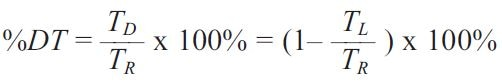(2)

Operating conditions that lead to more than 63% dead time should be avoided, wherever possible, due to escalation of the systematic errors in the dead time correction above that point. Also, most gamma-ray spectrometers integrate paralyzable dead time (also known as extending dead time) as the main component of their dead time. With paralyzable dead time, the maximum recorded counting rate is attained at 63% dead time. Above 63% dead time, the counting rate recorded in the analyzed spectrum1,2 is actually decreased when the counting rate is increased at the detector.

## Correcting for Dead Time Losses

The schemes for correcting for dead time losses can be classified into two main categories:

a) The counting rate of the source of gamma rays is essentially constant over the time taken for accumulating the spectrum
b) The counting rate varies considerably when the energy spectrum is accumulated

## The Live Time Clock for Constant Counting Rates

The most common situation is probably measuring the activities and energy spectrum of radioisotopes with half lives much longer than the time required for accumulating the energy spectrum with sufficient statistical precision. Here, the percent dead time and counting rate are essentially constant when the spectrum is measured. The livetime clock1,2 is a convenient and effective tool for correcting the dead time losses when the counting rates are constant. This is an electronic clock used for counting the ticks of elapsed time only when the spectrometer is live and able to respond to another gamma-ray event. This event resembles the measurement of the constant rate at which water is discharged by a pipe by filling a 1-liter bucket and then dumping the bucket on the ground. Dumping the bucket represents dead time. If the time taken for filling the bucket is only measured, the flow rate can be calculated in liters per seconds by dividing by the filling time. The time during which the bucket is emptied is not taken into account as it is the dead time. To get an answer with improved precision, this measurement can be replicated over a large number of 1-liter fillings, with the stop watch accumulating time during the filling phase, but stopped during the dumping time. The correct flow rate is derived at the end of the process by dividing the number of liters filled by the live time recorded on the stop watch.

Since a radioactive source generates the gamma rays with a random distribution in time, the number of gamma rays counted in a fixed time interval is a stochastic variable. The Poisson Statistics can be applied1 when the energy spectrum is accumulated for a preset live time (TL). Hence, the standard deviation in the recorded counts (NL) in a region of interest set across a specific peak in the spectrum will be1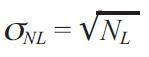(3)

The radioisotope’s activity is the subject of interest for gamma-ray spectrometrists and is proportional to the counting rate in the peak. The true counting rate at the detector is calculated free of dead time losses by dividing the recorded counts by the live time.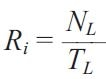(4)

The subscript on the counting rate (Ri) represents that this is the true input rate of gamma rays at the detector for the selected peak in the energy spectrum.

Here, the live time is assumed as a deterministically measured variable, and the standard formula for propagation of statistical errors3 provides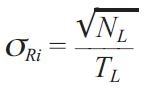(5)

Where,

σRi = The standard deviation in the counting rate (Ri)

Since the randomly arriving gamma rays generate the dead time intervals, there is a random variation in the ratio of TR/TL. As a result, the standard deviation in the number of counts recorded in a preset real time is not just the square root of the number of counts. Also, it is difficult to calculate the standard deviation in the counts recorded for a preset real time1,6,7. However, Equations (4) and (5) can be used irrespective of how the acquisition of the spectrum is stopped. In other words, the recorded counts should always be divided by the live time to obtain the true input counting rate, thus providing a simple way to derive the standard deviation in that counting rate.

The percent standard deviation in the measured input rate can be calculated using Equations (4) and (5) as follows: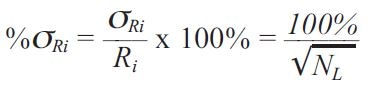(6)

How the precision from counting statistics relies on the number of counts recorded in a preset live time is shown in Table 1. Over 10,000 counts are required to attain a precision less than 1%.

Table 1. Percent Precision from Counting Statistics versus Live Time Counts.

NL % σRi
1
100
10,000
1,000,000
100
10
1
0.1

Refer reference 4 to know how this degrades when background needs to be subtracted from the peak in the energy spectrum. In this article, the background subtraction issue is ignored to simplify the discussion.

## Precision Limitations in the Livetime Clock

### Clock Tick Period

The electronic clock used to measure the live time and elapsed real time is usually based on a crystal-controlled oscillator that creates a period (tc) between clock ticks. The measured time is expressed in seconds by counting and multiplying the clock ticks by an appropriate constant. When a preset time limit is set, then the electronic clock ticks are counted to identify when that limit is reached to stop the acquisition.

The period (tc) needs be short compared to the pulse width caused by the gamma-ray event in order to accurately measure the dead time on each gamma-ray pulse. tc is typically designed to be in the range of 100 to 500 ns. An uncertainty of ±1/2 clock period in accounting for the actual elapsed time will be there when operating for a preset real time or a preset live time. In almost all cases, the preset time interval will be long enough (for example >20 ms) and as a result, this quantization error is negligible (<0.0025%).

### Truncation of the Reported Live Time

A second error can occur from the quantization limit set by the least significant digit in the live time as reported by the software or hardware. This precision limit is typically selected by design in the range of 10 ms to 1 s. If the spectrometer is run for a preset real time, the precision of the displayed live time is controlled by this quantization limit. If the live time is long in comparison with the least significant digit, this quantization limit contributes a negligible error to the counting rate measurement. However, a significant uncertainty can be introduced by the precision of the reported live time in the calculated counting rate when the elapsed live time for the measurement is nearing the magnitude of the least significant digit. In such cases, the live time precision becomes a limitation on the measurement precision. This limitation on the live time precision only applies when the acquisition is stopped manually, or when operating for a preset real time. This error can be eliminated by counting for a preset live time.

### The Systematic Dead Time Error

The third source of error gains significance when the percent dead time is very high. Pulse height discriminators are typically set just above the noise level for measuring the duration of the gamma-ray pulses to determine the dead time caused by each pulse. However, these pulses have falling and leading edges that are not very abrupt. The initial rise out of the noise is slow, and the falling edge is slow to disappear into the noise, thus making it difficult to accurately measure the dead time caused by each pulse. Low-energy pulses exhibiting a very low signal-to-noise ratio also pose a challenge for measuring their dead time contribution.

The following example will help understand why the accuracy of dead time measurement is significant at high percent dead times, but immaterial at low percent dead times. The measurement of the dead time interval is presumed to have a systematically high 0.01 relative error. The live time is 99% if the dead time is 1%. As a result, the dead time will be overestimated by 0.01 x 1% = 0.01%, which is a relative error of 0.01%/99% in the live time. This is roughly a 0.01% live time error, which will be a negligible value in comparison with the larger statistical error arising from counting random gamma-ray events.

At the other end of the range, a 90% dead time is considered, for which the corresponding live time is 10%. The 0.01 relative error in the dead time measurement leads to a 0.01 x 90% = 0.9% error in the total dead time. This is a relative error in the live time as expressed by 0.9%/10% = 0.09, which is a 9% error in the measurement of the live time. Here, the systematic overestimation of the dead time resulted in the systematic underestimation of the live time, and the corresponding overestimation of the counting rate by 9%.

Manufacturers often select a high percent dead time and specify an upper limit for the systematic live time error at that percent dead time. Generally, operating at a lower percent dead time will lead to an apparently lower live time error.

## ZDT for Varying Counting Rates

In gamma-ray spectrometry, applications with differing counting rates are sometimes encountered. Coolant pipe monitoring for contaminants in a nuclear power reactor is one such example. The counting rate will rise and fall abruptly when there is a brief surge in contamination. The analysis of samples activated by neutrons in a nuclear reactor is another example. The sample virtually always shows high counting rates from short-lived isotopes initially, followed by lower counting rates from radioisotopes that have much longer half-lives.

If there is a significant change in the counting rate during the time taken to collect the energy spectrum, a wrong counting rate will be yielded by the simple livetime clock. In neutron activation analysis, a high percent dead time is the result of the initially high counting rates and therefore, the spectrometer tries to compensate by increasing the counting time. However, the counting time is increased when the counting rate is low, thus distorting the spectrum. The counts for the short-lived isotopes will be systematically depressed and the long half-life content will be artificially increased.

This problem is overcome by the ORTEC Zero Dead Time algorithm5 with rapidly changing counting rates by performing the dead time corrections over time intervals that are small enough to experience any insignificant changes in counting rate.

### The Constant Counting Rate Approximation

To understand the method, the measurement of a source with a constant counting rate is considered. At the end of the preset live time, TL, the region of interest on a peak in the spectrum consists of a number of counts, Nu, which will be called the uncorrected counts. From the definition of real time and live time, the counting rate at the detector in the region of interest is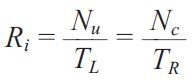(7)

Nc, the “corrected” counts, denotes the number of events that should have been recorded if there was no dead time. Nc can be calculated as follows using Equation 7: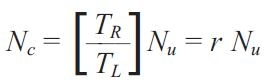(8)

Equation 9 more succinctly represents the real time to live time ratio in Equation 8 as follows: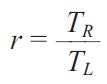(9)

The standard formula for the propagation of errors3 can be used to calculate the statistical variance in Nc as follows: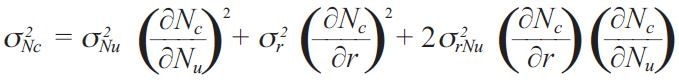(10)

Where,

The terms in parentheses are partial derivatives of Equation 8 with respect to the variables on the right side of Equation 8. (σrNu)2 is the covariance between r and Nu, and the statistical variance in r is (σr)2. Since the counts were obtained for a preset live time, the variance in Nu, is expressed as follows: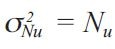(11)

For the case where the counting rate in the target region is a small fraction (f) of the total counting rate in the spectrum, Pommé6,7 has demonstrated that the second and third terms in Equation 10 are negligible and can be removed, thus simplifying Equation 10 to: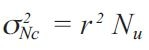(12)

Therefore, the standard deviation in the corrected counts is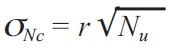(13)

If the counts in the integrated region of interest are not a tiny fraction of the total counts in the spectrum, the statistical error in Nc is underestimated by Equations 12 and 13. Practical measurements6 have shown that the standard deviation in the total spectrum counts can be as high as a factor of 1.45 higher than the value derived by Equation 13 when the percent dead time is 70%. Generally, a gamma-ray spectrometrist has interest in the activities of the radioisotopes as derived from the counting rates in the peaks. The counting rate in a specific isotope’s peak virtually always denotes a small fraction of the counting rate in the entire spectrum. Hence, Equation 13 can be applied in this normal case.

With this scheme for acquiring the corrected counts, the true input counting rate at the detector for the peak in the target region is expressed as follows: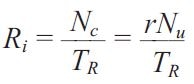(14)

It is to be noted that the corrected counts are divided by the real time to determine the true input counting rate. Equation 15 expresses the standard deviation in this calculated counting rate: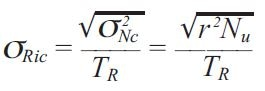(15)

## Adapting to Changing Counting Rates

If there is a significant change in the counting rates during the acquisition of the spectrum, it is not possible to obtain the right answer from Equations 7 through 9 and 11 to 15. This is due to the change in the real time to live time ratio (r) during the spectrum acquisition. At the end of the acquisition, the average value of r can be obtained. However, the average value of r2 required in Equation 12 and the square of the average value of r are not equal.

This problem is solved by the ZDT electronics by breaking up the acquisition into differential time intervals that are very short such that there are no significant changes in the counting rate during the differential interval. The duration of the differential time interval is typically selected by the design engineer to be in the range of 0.1 to 1.5 ms. This subdivision is based on real time to eliminate lengthening at high percent dead times.

The current ratio of real time to live time is measured during each differential time interval. When an analyzer accepts an event, instead of adding one count to the appropriate energy channel, the counts in that memory location are incremented by r counts, where ‘r’ is the instantaneous value of the ratio between real time and live time. As a result, the corrected counts are instantaneously generated from the uncorrected counts on each accepted event. Therefore, the correction can follow the changing values of r caused by the changing counting rates. At the end of the acquisition, the “Corrected” spectrum consists of the counts corrected in real time for the dead time loss. This spectrum indicates the spectrum of gamma-rays received by the detector over the real time of the acquisition, undistorted by dead time losses.

Equation 12 is then adapted to the differential time intervals to obtain the statistical variance in the corrected counts. The memory of the analyzer is classified into two equal segments. The first segment consists of the energy spectrum corrected for dead time losses and is called the “ZDT Corrected Spectrum.” The second spectrum is typically known as the “ZDT Error Spectrum,” which uses the same energy scale as the corrected spectrum. However, the numbers in each energy channel of the error spectrum denote the variance in the counts in the corresponding channel in the corrected spectrum.

The error spectrum is also produced on an event-by-event basis. The instantaneous value of r2 is included to the content of the appropriate energy channel in the error spectrum upon acceptation of a gamma-ray event by the analyzer. As can be derived from Equation 12, the variance for a single count is r2, because Nu = 1. The well known result for integrating independent random errors reveals that the variance of the sum of numbers is simply the sum of the individual variances3. Hence, the variance in the sum of the r values tallied in the corrected energy spectrum in the same energy channel is correctly reported when the individual values of r2 in the error spectrum are summed up.

In practice, one sets a target region across a peak in the ZDT corrected spectrum and adds the counts in that target region to report the corrected counts in the peak, Nc. The variances over the same channels in the ZDT error spectrum are summed up and the square root of that sum is taken in order to predict the standard deviation in the peak counts. In other words, the variance in Nc is: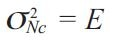(16)

Where, E represents the sum of the numbers in the ZDT error spectrum over the same channels used to sum the counts in the ZDT corrected spectrum. Apparently, the estimated standard deviation in the Nc counts is expressed as follows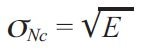(17)

It is to be noted that for completeness, the counting rate at the detector is determined by dividing the corrected counts by the elapsed real time, i.e.,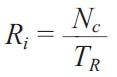(18)

and the standard deviation in the calculated Ri is derived from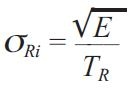(19)

Therefore, ZDT algorithms offer a means of correcting for dead time losses differentially in real time, with the ability to estimate the standard deviation in the corrected counts, even when the counting rates and percent dead time change significantly during the acquisition period. Pragmatic verification of the accuracy and effectiveness of the ZDT method is demonstrated by the extensive testing discussed References 6, and 8 through 13.

## Summary for Implementation

The following is the summary of the key points regarding the various operating conditions:

1. For counting rates that are essentially constant during the time taken for the acquisition of an energy spectrum
a) The calculated standard deviation in the recorded counts is the square root of the number of counts when acquiring for a preset live time. However, this statement does not hold good when acquiring for a preset real time.
b) The recorded counts are divided by the elapsed live time to determine the true counting rate at the detector (corrected for dead time losses). This step is applicable irrespective of how the acquisition is stopped (manually, by preset counts, by preset real time, or by present live time).
c) Irrespective of how the acquisition is stopped, the calculated standard deviation in the estimated counting rate from step ‘1b’ is the square root of the number of counts divided by the live time
2. The ORTEC ZDT mode can be used for rapidly changing counting rates, where the percent dead time changes considerably during the acquisition of the energy spectrum.
a) The counts corrected for dead time losses are determined by integrating the counts in a peak from the ZDT Corrected Spectrum
b) The variance in the counts from the peak is derived by summing the numbers in the ZDT Error Spectrum over the same channels employed for summing the counts in the peak. The estimated standard deviation is simply the square root of the variance summed from the ZDT Error Spectrum.
c) The counting rate at the detector (corrected for dead time losses) is the corrected counts from step 2a divided by the elapsed real time
d) The estimated standard deviation in the counting rate at the detector is the standard deviation from step 2b divided by the elapsed real time
3. To avoid digital truncation errors and systematic errors in the livetime correction
a) Use total acquisition times that are larger than the reported least significant digit from the livetime clock
b) Avoid percent dead times in excess of 63% wherever possible

## References

1. Ron Jenkins, R. W. Gould and Dale Gedcke, Quantitative X-Ray Spectrometry, Marcel Dekker Inc., New York, 1981, Chap. 4. [Ed., The 1st edition is recommended, because relevant information beyond the middle of section 4.8 has been deleted in the 2nd edition, and the graphics for figures 4.55 and 4.56 have been erroneously interchanged.]

2. See the Introduction to Amplifiers and the Introduction to CAMAC ADCs on the ORTEC web site, www.ortec-online.com.

3. Phillip R. Bevington and D. Keith Robinson, Data Reduction and Error Analysis for the Physical Sciences, WCB/McGraw-Hill, New York, 1969.

4. D. A. Gedcke, ORTEC Application Note AN59, How Counting Statistics Controls Detection Limits and Peak Precision, www.ortec-online.com, 2001.

5. Russell D. Bingham, Dale A. Gedcke, Rex C. Trammell, Timothy R. Twomey and Ronald M. Keyser, Differential Correction Method and Apparatus, U. S. Patent No. 6,327,549 B1, Dec. 4, 2001.

6. S. Pommé, Nucl. Instr. and Meth. In Phys. Res. A, 474 (2001) 245–252.

7. S. Pommé, Nucl. Instr. and Meth. In Phys. Res. A, 482 (2002) 565–566.

8. D. Upp, R. Keyser, D. Gedcke, T. Twomey, and R. Bingham, An Innovative Method for Dead Time Correction in Nuclear Spectroscopy, Journal of Radioanalytical and Nuclear Chemistry, Volume 248, Number 2/May, 2001, pp 377–383

9. R. Keyser, D. Gedcke, D. Upp, T. Twomey, and R. Bingham, A Digital Method for Dead Time Compensation in Nuclear Spectroscopy, (Presented at the 23rd Brugge ESARDA meeting on Safeguards and Nuclear Materials Management, May 2001), Proceedings of the ESARDA 23rd Annual Meeting : Symposium on Safeguards and Nuclear Material Management, Brügge, 2001. – 2003. – (EUR-19944.EN). – 92-894-1818-4. pp 666–671

10. D. Gedcke, R. Keyser, and T. Twomey, A New Method for Counting Loss Correction with Uncertainty in Gamma Spectroscopy Applications, Proceedings INMM 42nd annual meeting, Indian Wells, CD-ROM, 2001, http://www.orteconline.com/papers/inmm_zdt.pdf.

11. R. Keyser, T. Twomey, and R. Bingham, Performance of the Zero-Dead-Time Mode of the DSPEC Plus, Proceedings of the 2001 Annual ANS Meeting, June 17, 2001, Trans. Amer. Nucl. Soc., Vol 84, PBD

12. R. Keyser, T. Twomey, and D. Upp, PerkinElmer Instruments-ORTEC, and R. Sillanpaa, Teollisuuden Voima Oy, Analysis of Long-lived Isotopes in the Presence of Short-Lived Isotopes Using Zero Dead Time Correction, Nuclear Science Symposium Conference Record, 2001 IEEE Vol. 2, 4 – 10 Nov. 2001, pp 725–727, http://www.orteconline.com/papers/analysis_long_lived.pdf.

13. ORTEC Application Note AN56, Loss Free Counting with Uncertainty Analysis Using ORTEC’s Innovative Zero Dead Time (June 2002)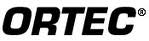This information has been sourced, reviewed and adapted from materials provided by ORTEC.

## Citations

• APA

ORTEC. (2019, May 01). Managing Dead Time Errors in Gamma-Ray Spectroscopy. AZoM. Retrieved on July 10, 2020 from https://www.azom.com/article.aspx?ArticleID=13914.

• MLA

ORTEC. "Managing Dead Time Errors in Gamma-Ray Spectroscopy". AZoM. 10 July 2020. <https://www.azom.com/article.aspx?ArticleID=13914>.

• Chicago

ORTEC. "Managing Dead Time Errors in Gamma-Ray Spectroscopy". AZoM. https://www.azom.com/article.aspx?ArticleID=13914. (accessed July 10, 2020).

• Harvard

ORTEC. 2019. Managing Dead Time Errors in Gamma-Ray Spectroscopy. AZoM, viewed 10 July 2020, https://www.azom.com/article.aspx?ArticleID=13914.

## Tell Us What You Think